This project was inspired by the idea of particle behavior out of a discussing of the COVID-19 pandemic. The idea of creating a particle system that is able to converge and disperse was chosen as the exercise to develop the understand of python and grasshopper’s capabilities.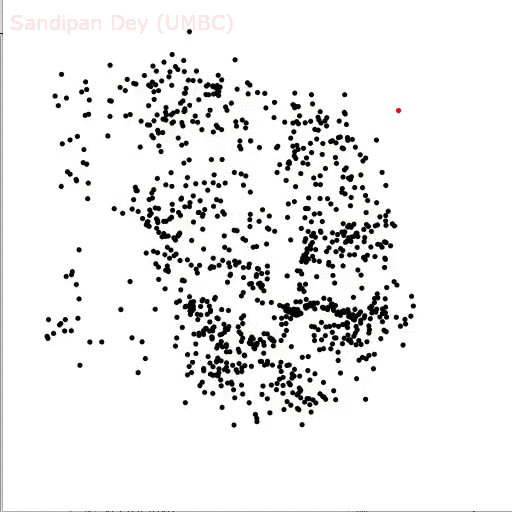As a starting point, the flocking boids simulator is implemented with 2-d-trees and shows how the flock of birds fly together, the black / white ones are the boids and the red one is the predator hawk. The jelly float simulation shows the movement of points in relation to the movement a pre-formed gelatin-like geometry.

##### BUILDING A SYSTEMIn order to create a system of particles, several rules can be layered together to create behaviors. The following are the rules most relating to the idea of the concept of this project. Separation, alignment, and cohesion were studied as  potential the behaviors that could mimic a sentient, virus-like particle.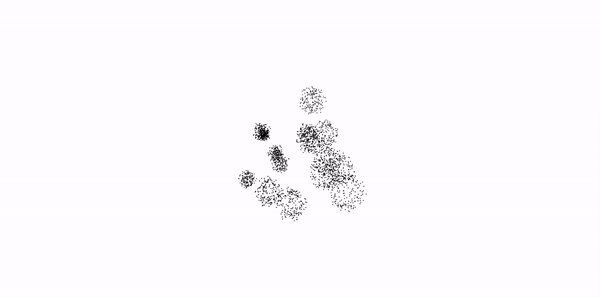The first GIF displays separation, cohesion, and spin force. Each particle has a starting point and uses spin force to generate an initial movement. The particles then start to come together using cohesion, as the particles approach a specified minimum distance they separate which then increases their spin force. The second GIF displays the the particles as particle communities to visualize the behavior using larger volumes.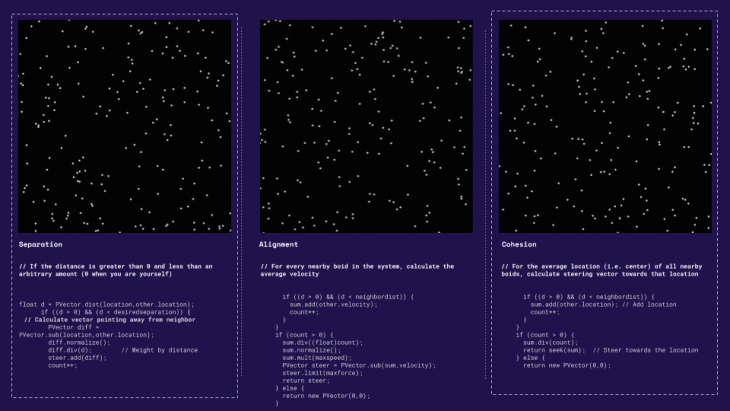Separation // If the distance is greater than 0 and less than an arbitrary amount. Cohesion // For the average location (i.e. center) of all nearby boids, calculate steering vector towards that location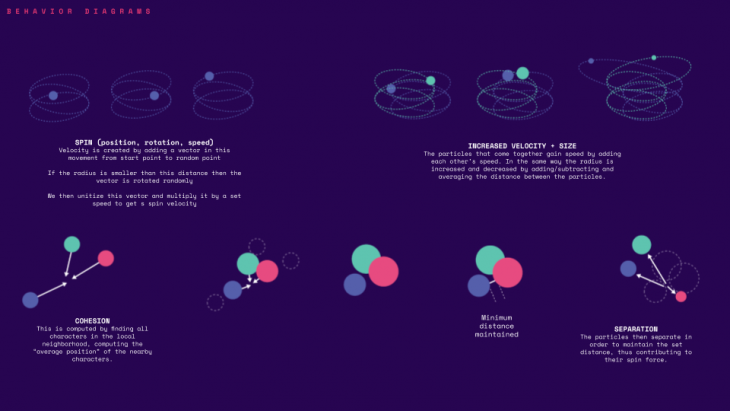The image above displays the steps taken to add a third behavior of changing particle sizes using velocity. Velocity is created by adding a vector in this movement from start point to random point. If the radius is smaller than this distance then the vector is rotated randomly. We then unitize this vector and multiply it by a set speed to get s spin velocity.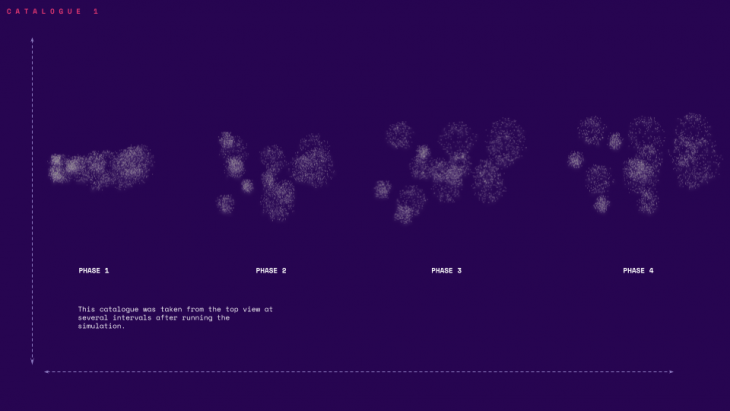Increasing Velocity and Size // The particles that come together gain speed by adding each other’s speed. In the same way the radius is increased and decreased by adding/subtracting and averaging the distance between the particles.

##### PYTHON STRING + GRASSHOPPER SCRIPT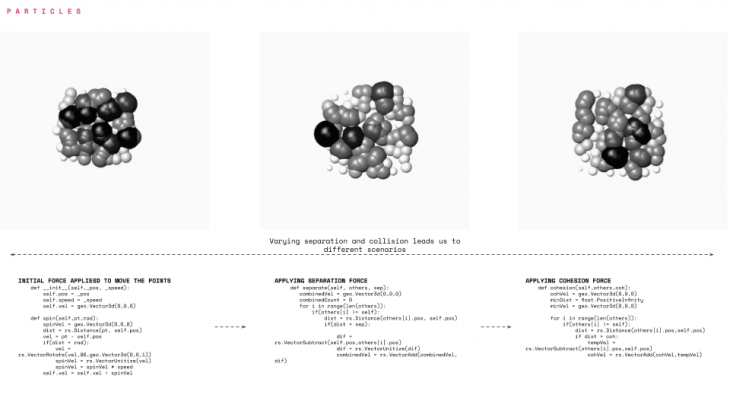The image above illustrates the string used to develop each behavior along with the grasshopper script showing the post production of the particles. The final step was the addition of post-visualization of the particles.

##### VISUALIZATIONS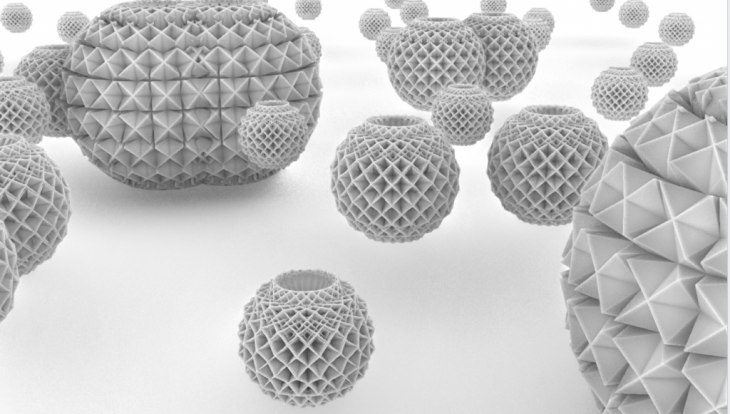This visualization displays the particles closer to the identity of a virus using mesh subdivision.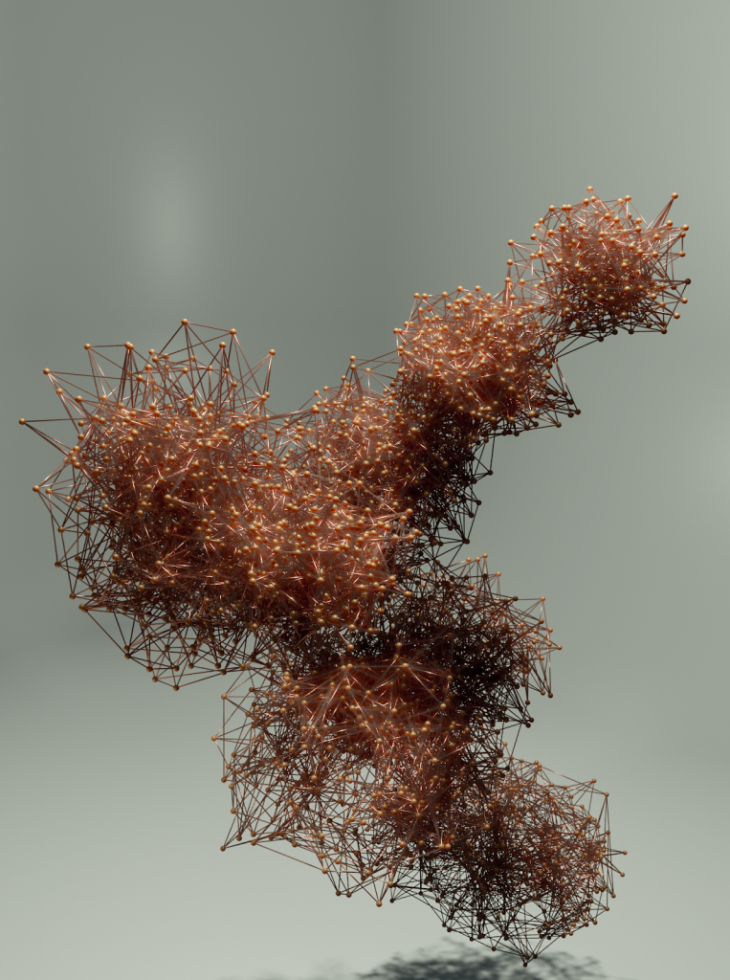The above images split the mesh into points and draw lines connecting each particle and particle community.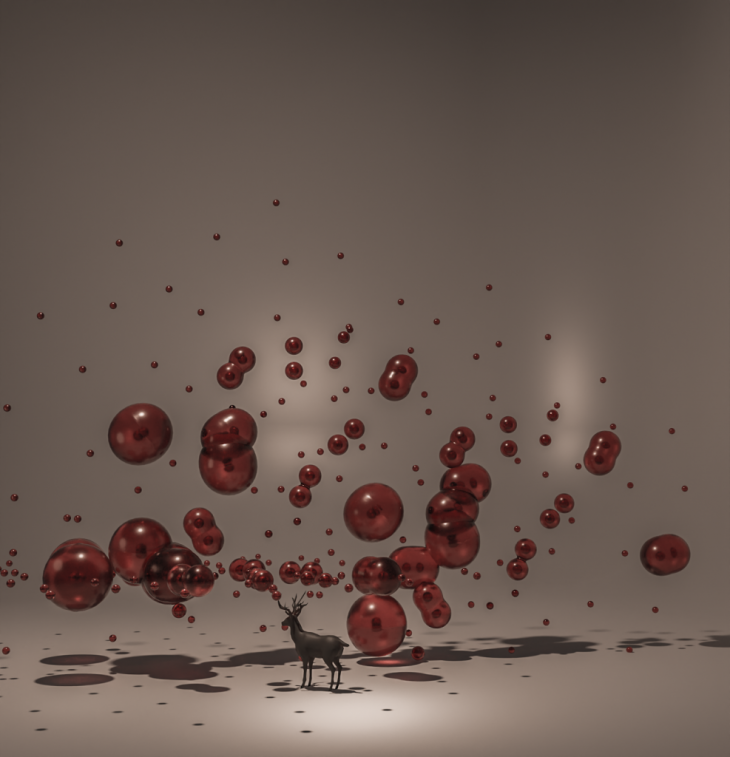The final visualization rendition displays the cohesion limitations using a merging mesh face.